# Plums

In the bag was to total 136 plums. Igor took 3 plums and Mary took 4/7 from the rest. How many plums remained in the bag?

x =  57

### Step-by-step explanation: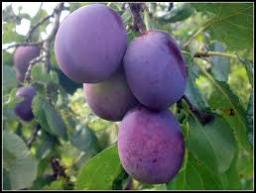Did you find an error or inaccuracy? Feel free to write us. Thank you!Tips to related online calculators
Need help to calculate sum, simplify or multiply fractions? Try our fraction calculator.
Do you have a linear equation or system of equations and looking for its solution? Or do you have a quadratic equation?

## Related math problems and questions:

• Rope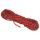Half of the rope used Mam for packaging, half of the rest got a son, half of the rest he took the father and two-fifths of what remained, and got a daughter. Remained 143 cm of rope. How long was the rope at the beginning?
• Resort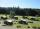In a completely crowded resort was 30% from the Czech Republic quarter visitors were from Slovakia and the rest 135 came from Germany. What capacity have resort?
• OrchardOne-eighth of the trees in the fruit plant in winter froze, and one-twelfth of damaged disease and pests. Healthy trees remained 152. Is it enough to supply 35 trees to restore the original number of trees in the orchard?
• Dividing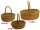When dividing the tangerines into packages of 8 or 10, one always remained. How many were there if there were more than 250 and less than 300?
• How manyHow many integers are greater than 547/3 and less than 931/4?
• The balls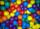You have 108 red and 180 green balls. You have to be grouped into the bags so that the ratio of red to green in each bag was the same. What smallest number of balls may be in one bag?Convert magnitude of the angle α = 136°18'10" to radians:
• FruitsAmy bought a basket of fruits 1/5 of them were apples,1/4 were oranges, and the rest were 33 bananas. How many fruits did she buy in all?
• PlumsIn the bowl are plums. How many would be there if we can divide it equally among 8, 10 and 11 children?
• Magic bagEach time the prince crossed the bridge, the number of tolars in the magic bag doubled. But then the devil always conjured 300 tolars for him. When this happened for the third time, the prince had twice as much as he had in the beginning. How many tolars
• Shop stores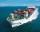Workers untied fruit to stores. In the first quarter shipments, in the second fifth shipment, in the third two-fifths of the rest and in the fourth 231 kilograms. How many fruits were together?
• Camp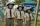In the camp are children. 1/2 went on a trip, 1/4 went to bathe, and 38 children remained in the room. How many children are in the camp?
• There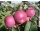There are 32 trees of three species in the orchard. 25% cherries, three eighty pears, others plums. How many plums are in the orchard?
• Plums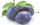By drying 3 kg of fresh plums, we obtained 750 g of dried plums. How many kg of fresh plums need to be dried if we want to get 1.5 kg of dried plums?
• Divisible by 5How many three-digit odd numbers divisible by 5, which are in place ten's number 3?
• Mixing operations with numbersSubtract twice the number -23.6 from the difference of the numbers -130 and -40.2.
• Elections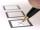The elections attended a total 4818 voters. First day 39 men more than women, the second day 21 women more than men. How many women attended elections?